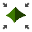The Rocscience International Conference 2021 Proceedings are now available. Read Now

# Scale Wedge

The Scale Wedgeoption in RocPlane allows you to scale the size of wedges according to Joint or Tension Crack Persistence, Wedge Volume, or Wedge Weight.

To use the Scale Wedge option:

1. Select Scale Wedgeon the Analysis menu.
The Scale Wedge dialog appears showing the current wedge parameters.2. Select the check box(es) for the scaling parameters you want to adjust (Maximum Persistence, Volume, Weight, etc.) and enter the desired Scaling Values .
3. Click Apply or OK to re-compute the analysis using the scaled wedge size.
All analysis results will now apply to the scaled wedge size.

## Scaling Parameters

### MAXIMUM PERSISTENCE

The Maximum Persistence refers to the two-dimensional length of the joint Failure Plane or Tension Crack plane, as shown in the figure below.RocPlane definition of Joint Persistence is the two-dimensional trace of the wedge failure plane

### WEDGE VOLUME / WEIGHT

Wedge size can be scaled according to Wedge Volume or Wedge Weight. Note that these parameters are directly related to the rock Unit Weight (i.e., Wedge Weight = Wedge Volume * Rock Unit Weight). In addition, Wedge Volume and Wedge Weight must be specified per unit weight of slope.

### MULTIPLE SCALING VALUES

If multiple scaling parameters are entered in the Scale Wedge dialog, only the scaling parameter that produces the smallest weight is applied. All other scaling values are ignored.

## Probabilistic Analysis and Scaling

In a Probabilistic analysis, multiple scaling parameters can be useful if the wedge plane orientations are random variables because different scaling parameters can be applied to different wedges. However, for any individual wedge generated by a Probabilistic analysis, only the scaling parameter that gives the smallest wedge is applied.

NOTE: The Scale Wedge option cannot be used in conjunction with the Persistence Analysis option. If the Persistence Analysis option is in use, the Scale Wedge option is not available, and vice-versa.